# Flo-maps fractograms: the prequel

Hugh Duncan returns with the long-awaited prequel in which he further explores the geometric patterns hidden behind the fractions.My original interest in decimal fractions was due to studying the ‘chaotic dropper’ experiment (see Fractograms from Chalkdust issue 02). Long before this, I had read about modelling the growth of a population and finding that it, too, can demonstrate chaotic behaviour. This is shown by using a logistic map. I realised the digits of decimal fractions could be subjected to the same modelling process. Let me explain.

## The logistic map

A logistic map is a graph that shows how certain types of modelling functions vary with time. The best example to demonstrate this is a function for the growth of a population. It is based on the idea that if a population has space to grow and food to eat, it will increase in number. If the population becomes overcrowded or runs low on food, its numbers will fall. The model starts at time $n = 0$ with a certain population, say $x_0$, which is normalised by setting it to a value between $0$ and $1$. The maximum ‘$1$’ means saturation and the animals are squished together like people in an overcrowded lift. Zero means, well, like the Dodo: extinct. To find the new population, $x_1$, at time $n = 1$ (the next moment or breeding season), the following function is applied:

$$x_{n+1} = ax_n(1 – x_n).$$

Here, $a$ is a coefficient: the rate at which the population grows. If $a$ is small (close to zero), the population grows slowly, but if it is large (up to $4$ max), the population will breed like rabbits! The $(1-x)$ term indicates how much free space is left, into which the population grows. Less space to grow into means less growth is possible. This makes the value of $x_1$

$$x_1 = ax_0(1-x_0).$$

To find the next population $x_2$ at $n = 2$, the population at time $n = 1$ is subjected to the same process:

$$x_2 = ax_1(1-x_1).$$

This process can be iterated over time. For example, take a starting population of, say $x_0 = 0.2$ and $a = 2$ and we get:

\begin{align*} x_1 &= 2 \times 0.2 (1-0.2) = 0.4 \times 0.8 = 0.32\\ x_2 &= 2 \times 0.32 (1-0.32) = 0.64 \times 0.68 = 0.4352\\ x_3 &= 2 \times 0.4352 (1-0.4352) = 0.4916…\\ x_4 &= 2 \times 0.4916(1-0.4916…) = 0.49986… \\ & \text{ the population approaches 0.5.}\end{align*}

Here comes the logistic map. We plot the value of $x$ against these time increments. This shows how the population, $x$, changes with time, $n$. Notice the population grows fast at first but gradually slows its growth rate to reach a steady, stable total. Our ‘animals’ are in equilibrium with their environment—not too crowded, not too sparse—and the death rate balances the birth rate.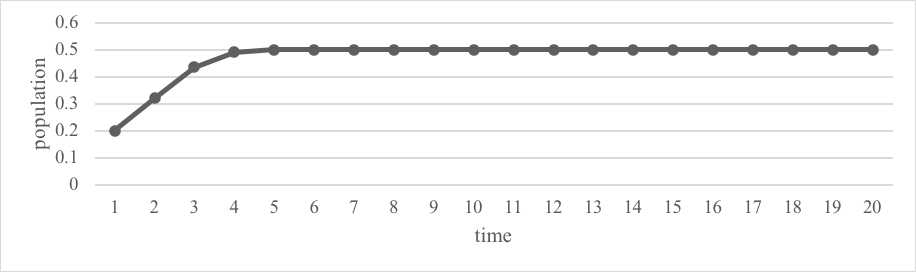Logistic map corresponding to $x_0 = 0.2,\ a = 2$.

Now let’s heat things up a bit. Keeping the same starting population $(0.2)$, but raising the growth rate to $a=3$. We observe that the population grows much faster at the beginning, but then oscillates between two values.Oscillating value of population obtained from setting the growth rate to $a = 3$.

The population overshoots and due to ‘overcrowding’, the numbers drop, but as there’s enough space now, it overgrows again and there it oscillates indefinitely between these two values. Real populations actually do this, such as rabbits, which overbreed, then there’s not enough food so they starve and the population goes through an up-down variation. The foxes that eat the rabbits also vary in the same way but slightly out of step as their food source rises and falls.

Now if $a = 3.53$, the population tends to oscillate between four values. The logistic map is:Oscillating value of population obtained from setting the growth rate to $a = 3.53$.

Finally, let $a = 3.8$ and see what unfolds. Look below and see and what was always, up until now, coming to a happy, predictable ending is now quite the opposite: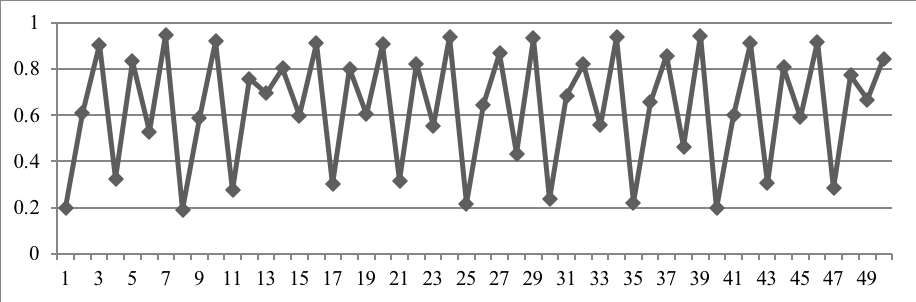Evolution of population corresponding to $a = 3.8$. We observe chaotic behaviour.

The logistic map suggests that, under certain conditions, a population that obeys simple rules of evolution, can display what is known chaotic behaviour.

## The fraction logistic map-cycle one

Let us use the logistic map as an analogy to study the structure of repeating decimal fractions.  For simplicity, let us take a fraction with the same repeating digit, such as $8/9 = 0.88888888\dots$ For the representation of the fraction, the $x$-axis will show the position value, that is, the tenths, hundredths, thousandths and so on, while the $y$-axis will give the actual value of the digit itself in that position, 0, 1, 2 etc up to 9. The coordinates in the case of $8/9$ would be $(1,8)$, $(2,8)$, $(3,8)$ and so on. This fraction logistic map or flo-map as I call it is:Flo-map of the fraction $8/9$.

Not surprisingly, the graph shows a horizontal line, as each digit has the same value as the previous. This flat-lining fraction is mimicking that part of the population logistic map where it is stable. Any fraction of the form $n/9$ where $n$ is an integer from 1 to 8 will fall into this same behaviour.

## The two-figure logistic map

The next simplest repeating fraction has a recurring behaviour involving two figures. The  unit fraction with the smallest denominator in this category is $1/11 = 0.090909…$. The coordinates would be $(1, 0)$, $(2, 9)$, $(3, 0)$, $(4, 9)$ and so on. This is shown below: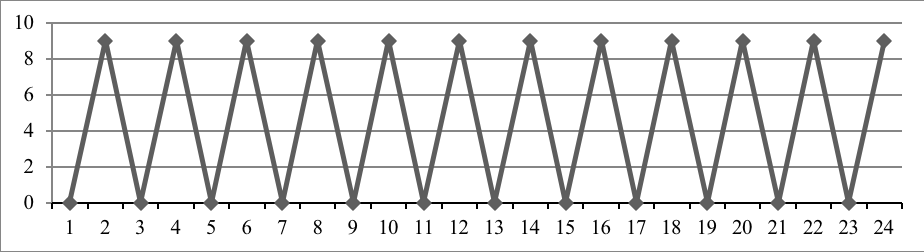Flo-map of the fraction $1/11$.

A bit more interesting, this graph jumps up and down between $0$ and $9$ and mimics that part of the population logistic map that oscillates between two values. Any fraction whose decimal expression has two recurring figures will fall into this category.

## King fraction the seventh

Let us now use the logistic map to study the structure of my favourite repeating decimal fraction, that of $1/7$. The fraction $1/7$ as a decimal is $0.142857 142857…$ as we saw above: a repeating pattern involving $6$ figures. It is plotted on the logistic map here:Flo-map of the fraction $1/7$.

The repeating cycle is apparent. Each fraction has its own ‘signature’ and they are quite varied and distinctive. I wanted to share just one more example: that of $1/9801$ (below). Isn’t it curious?! It repeats every 199 digits and looks like a piece of modern art!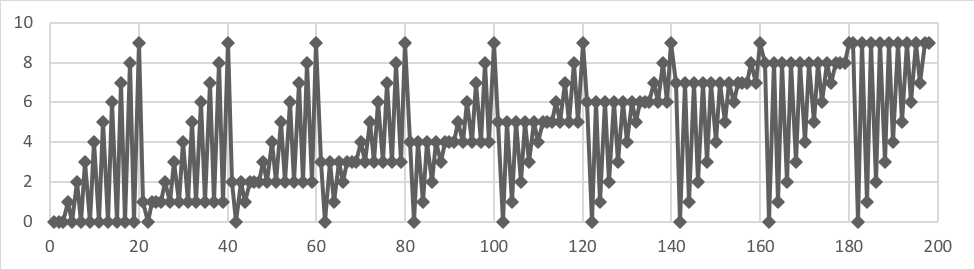Flo-map of the start of $1/9801 = 0.00010203040506…$.

## Engineered fractions

By a suitable choice of digits, one can make almost any regular repeating line that cannot double back on itself. I called these engineered fractions. The graph below shows what I call the sinusoidal curve. The digits of this decimal fraction are $0.013689\,986310\,013689\,986310…$ This repeating series turns out to be the digits of the fraction $1369/1000001$. It is not quite a true sine curve, but it looks close enough! Many other shapes have been found, like a square wave, a staircase and ones that look like meerkats to name a few. There will be a chance to discover them and others at your leisure with an interactive flo-map maker shown below!The sinusoidal curve $1369/1000001$.

## The irrational and transcendental numbers

As irrational and transcendental numbers have no repeating pattern in their decimal expansions, one would expect to see a flo-map that looks completely random. If we check out the digits of the transcendental number $\pi$, we will not be disappointed: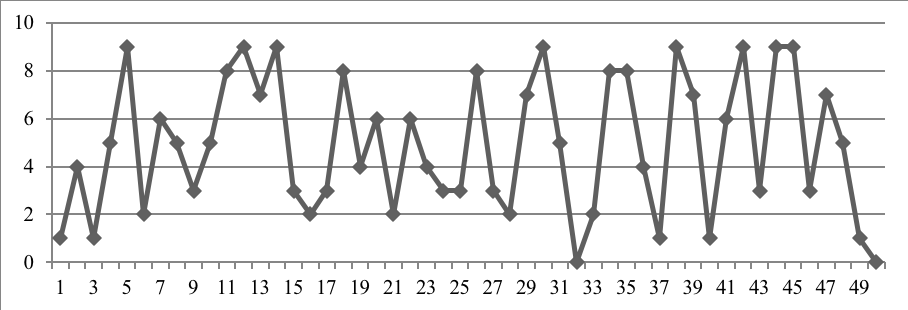Flo-map of $\pi = 3.14159365358979323846264338327…$

I think you’ll agree that it looks fairly random. It won’t surprise you to know that the flo-maps of numbers like $\sqrt{2}$ and $e$ are similarly random-looking.

## The superposed fraction logistic map

The superposed flo-map puts all the fractions with the same denominator onto the same logistic map. The first one that came to mind is $1/7$ with all those that follow it: $2/7$, $3/7$ up to $6/7$ (which collectively I call $+1/7$, the plus sign meaning it contains the complete set of fractions, as used in the original fractograms article). What makes this one worth checking out is that each decimal version contains the same $6$ repeating digits in the same order, but starting at a different place. To spell it out:

\begin{align*}1/7 &= 0.142857… \\ 2/7 &= 0.285714… \\ 3/7 &= 0.428571… \\ 4/7& = 0.571428… \\ 5/7 &= 0.714285… \\ 6/7 &= 0.857142…\end{align*}

Plotting all these digits onto the same graph, joining the dots and repeating several cycles, one gets the pattern below. It looks like a frieze, the border to a piece of artwork or a Persian carpet.The superposed $+1/7$ flo-map.

And as a parting shot, why not look at the not-so-seemingly-exciting $1/11$ and all its family members? Well, they are each only a two-figure repeating fraction, $1/11 =0.0909$, $\ 2/11 = 0.1818$ and so on, but put them all together and you get:The superposed $+1/11$ flo-map.

Isn’t that cool?! The separate zigzags have become a chain of diamonds like Christmas decorations! It shares the same properties as the others, with the $4.5$ line symmetry and the one-digit size copies and yet is quite distinctive and different to the others we have looked at so far.

## The series flo-map and digital music

The number $1/101$ is a four-figure recurring fraction: $(0.0099 0099 \text{ etc})$ as is $2/101: (0.0198\ 0198\text{ etc})$ and so on. I wondered what a flo-map of the four digits of each fraction would look like placed consecutively: that is $0099\,0198\,0297\,0396 ….$.  See the first 40 or so digits here:The superposed flo map of $+1/101$.

Seeing the points arranged like this and seeing it was a four-figure pattern, it reminded me of a ‘four beats to the bar’ piece of music! And I said unto myself: ‘Let zero be ‘C’, one be ‘D’ and so on for all ten digits and notes, up to the C and D above middle C. And, lo, it was all good. The whole sequence of the digits of $1/101$ to $100/101$ could represent a tune in 4/4 time (400 notes in all). I put the challenge to my musical friend Penny and her (at the time) ten-year-old musical/IT son Xavier and they turned the digits into a tune: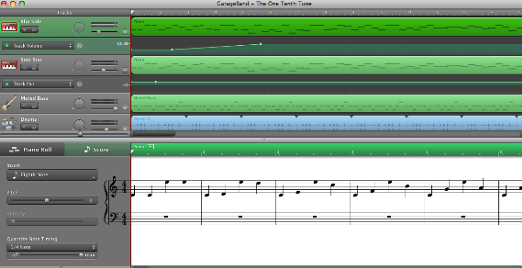The $+1/101$ song.

Listen to it here!

One could argue the creative merits of such a tune but I have likened it to a gentle kind of robot’s ‘lift music’. I wonder what other curious tunes lurk among these fractions? I would like to thank student Xavier Gilli and his mother Penny Mac McMorris (singer / musician / recording artist / songwriter / composer of musical theatre at Archangel Music Creatives) for their help in turning maths into music.

## The program

Due to the success of the game of life program in my earlier Reproduce or die article, my now graduated student Dmitry Mikhailov has kindly created another interactive program (based on a program by my son Fabien), to produce flo-maps of recurring fractions. It can be found below. Hopefully it is self-explanatory but here is a quick summary: input the numerator an integer, eg 1, then the denominator, eg 19, then select the number of decimal places, eg 50, which will cover a few cycles in this case, then ‘go’ and you should see the flo-map for $1/19$. By inputting again, it will replace the previous graph. I would start with small numbers first then experiment with larger ones. Displaying more than a few hundred decimal places might appear saturated and lose some visual beauty so use your own judgement. Go on, have a go, fractions speak louder than words!Hugh Duncan graduated from UCL having studied astronomy. He taught physics and maths at the International School of Nice for over thirty years. Hugh was a punk when he started UCL in 1976 and guess what, he still is! Check out his band Old Age Spies on YouTube. He recently retired and has just had his first science fantasy book published ‘Life On Mars – The Vikings Are Coming’ inspired by his time at UCL. Check it out too!